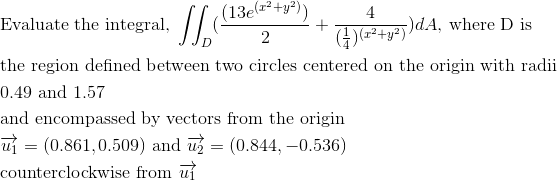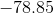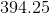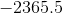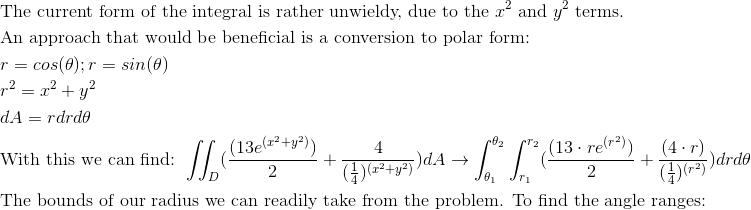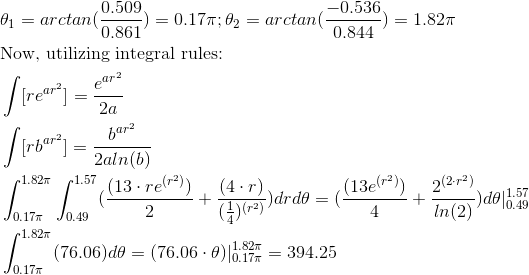Calculus 3 : Double Integrals

Example Questions

Example Question #61 : Double Integration In Polar Coordinates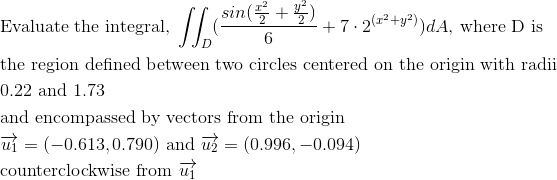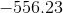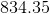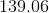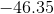Explanation: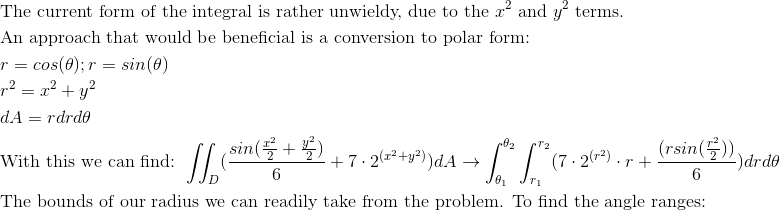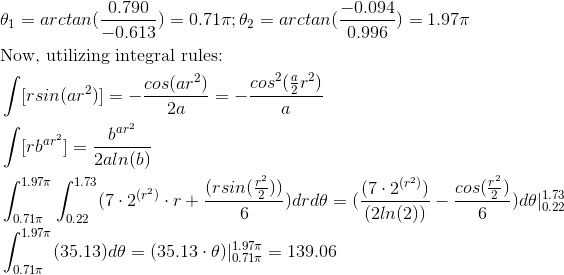Example Question #62 : Double Integration In Polar Coordinates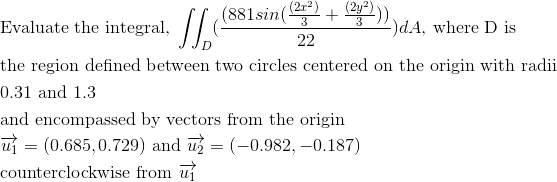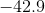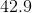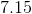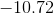Explanation: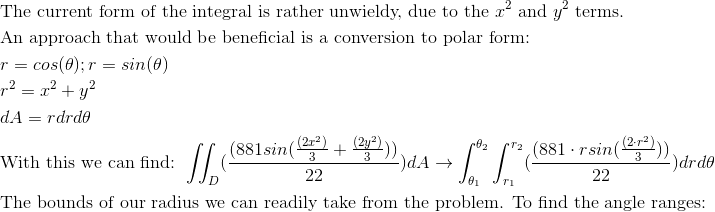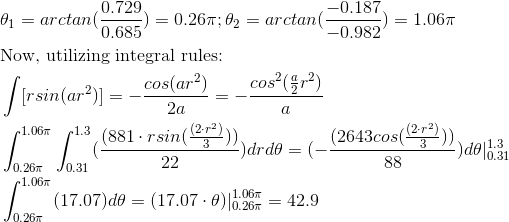Example Question #63 : Double Integration In Polar Coordinates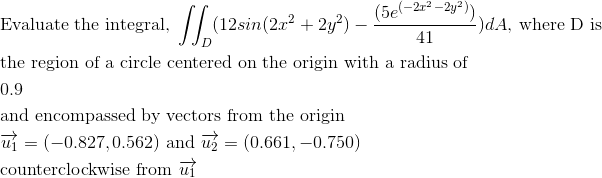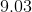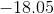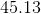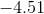Explanation: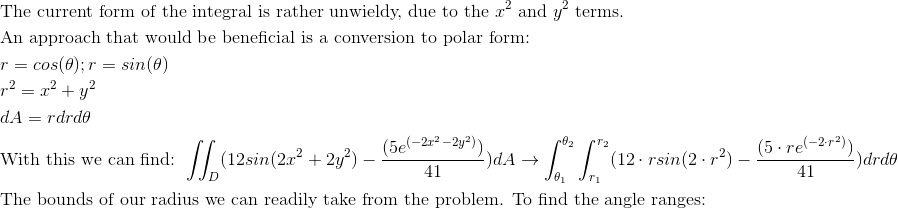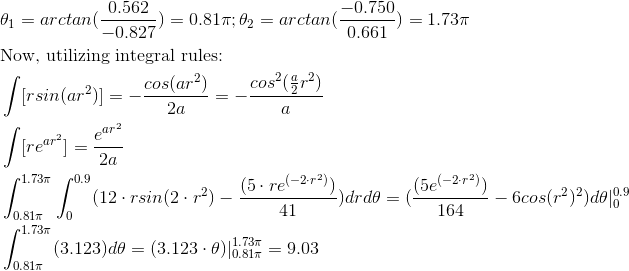Example Question #64 : Double Integration In Polar Coordinates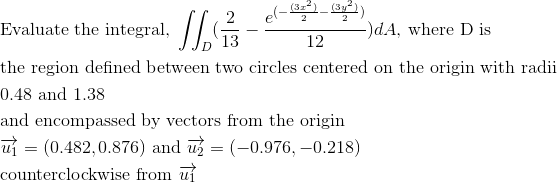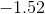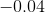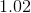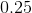Explanation: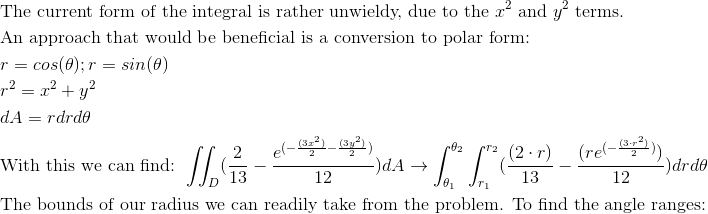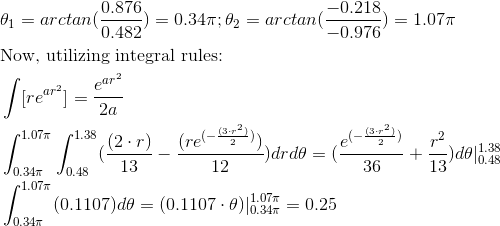Example Question #65 : Double Integration In Polar Coordinates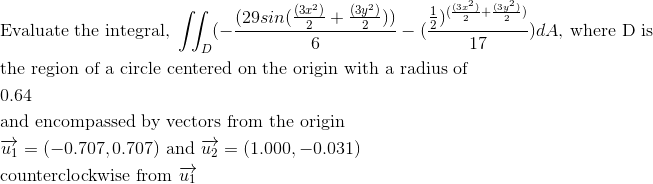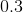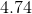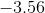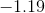Explanation: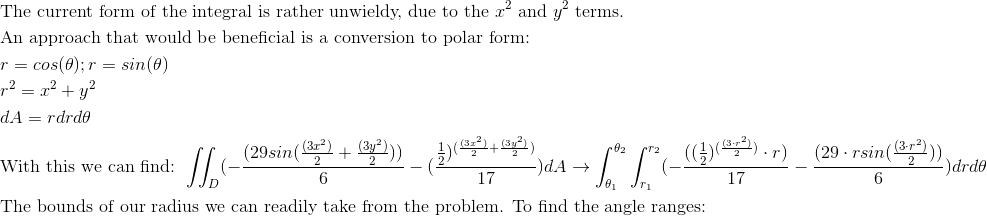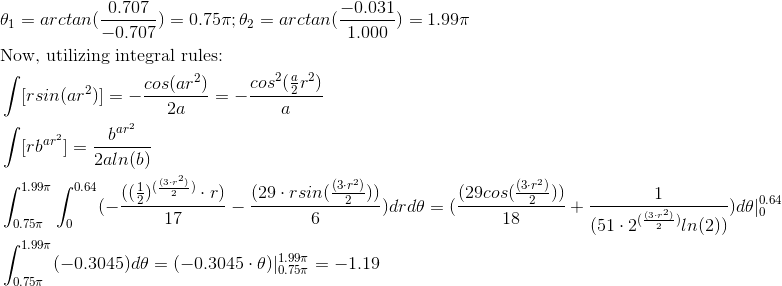Example Question #66 : Double Integration In Polar Coordinates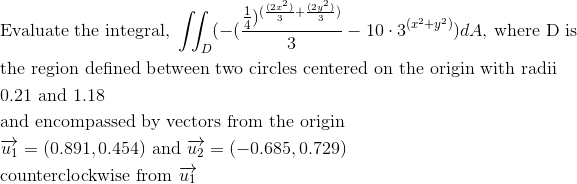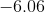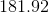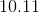Explanation: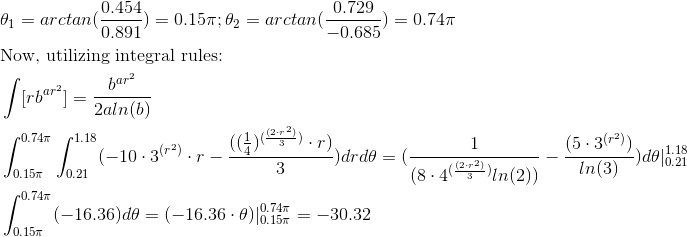Example Question #67 : Double Integration In Polar Coordinates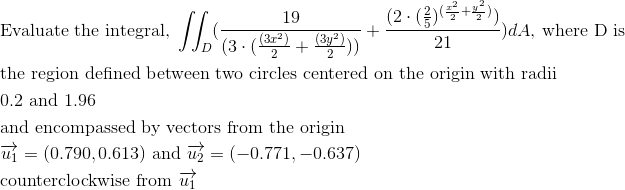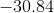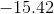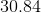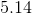Explanation: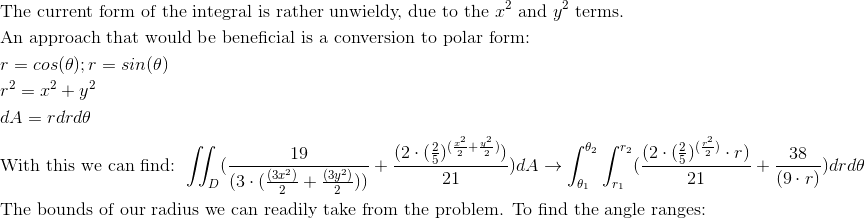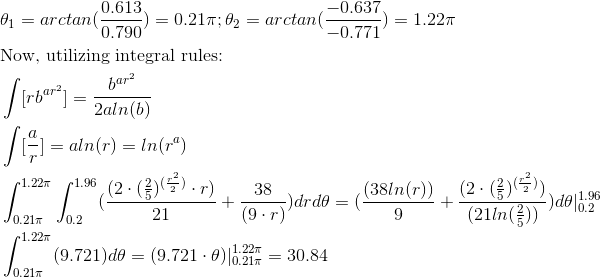Example Question #68 : Double Integration In Polar Coordinates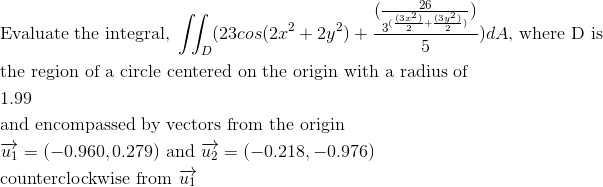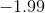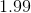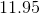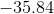Explanation: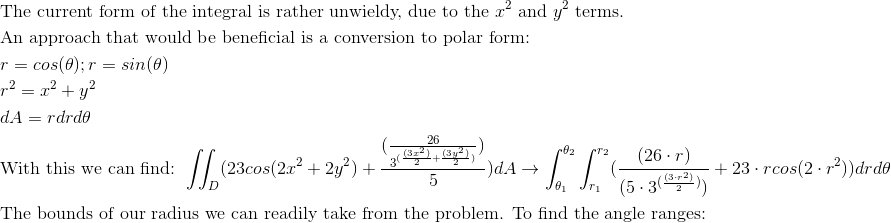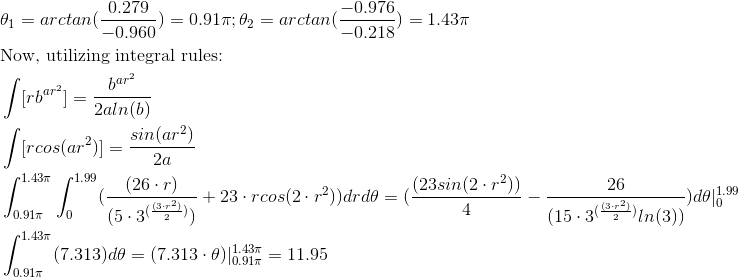Example Question #69 : Double Integration In Polar Coordinates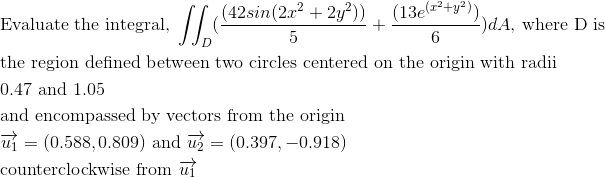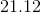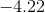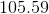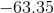Explanation: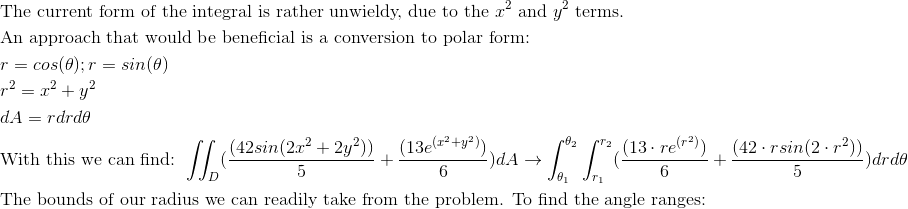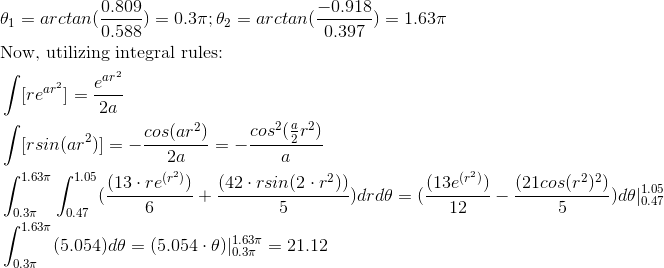Example Question #70 : Double Integration In Polar Coordinates